# Fosdem2017 Scientific computing on Jruby

Feb. 4, 2017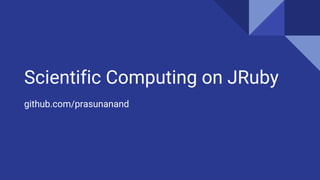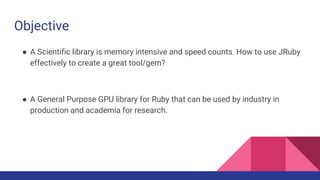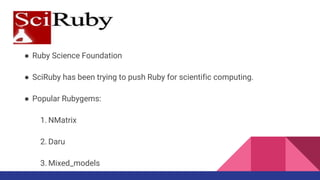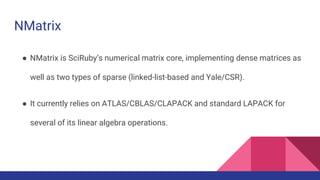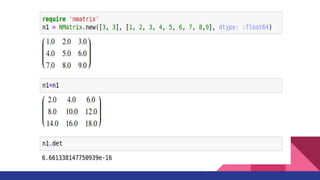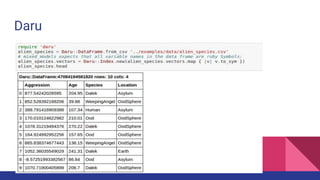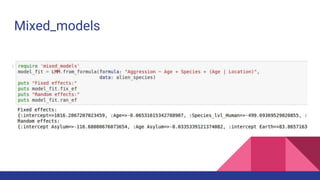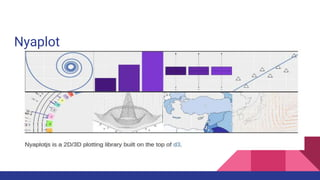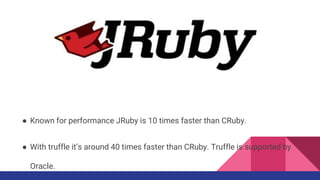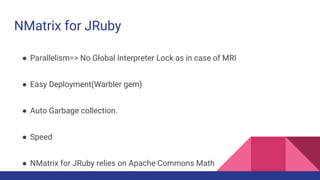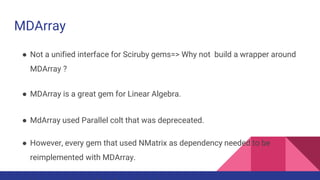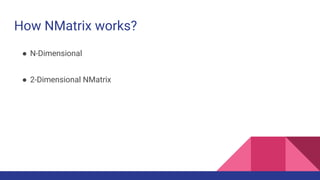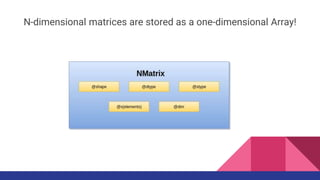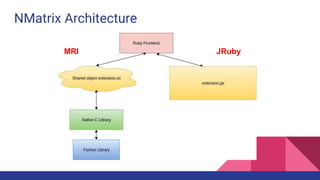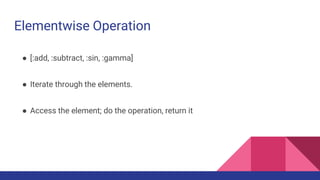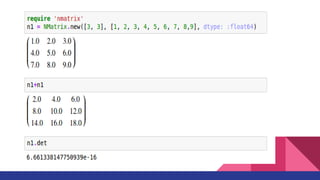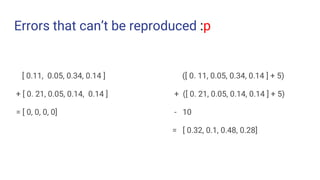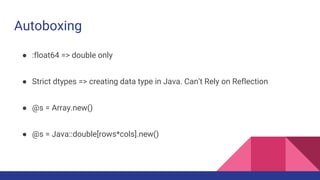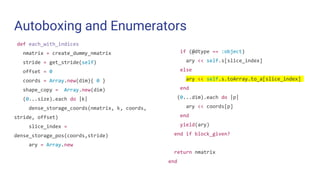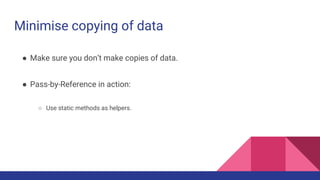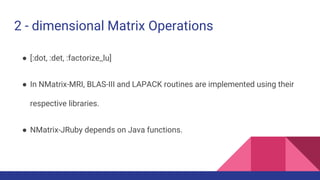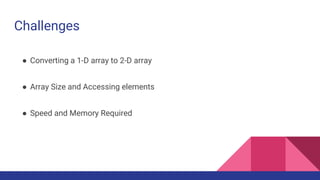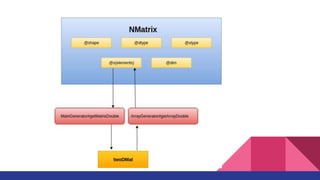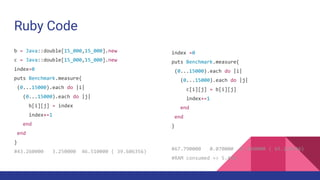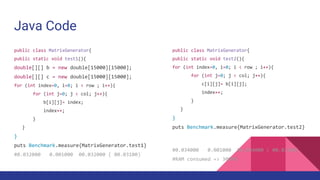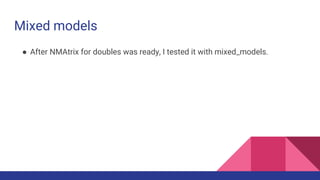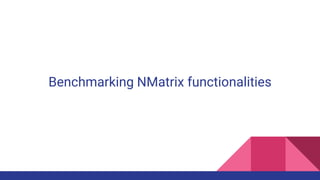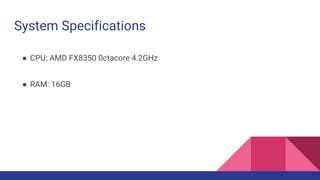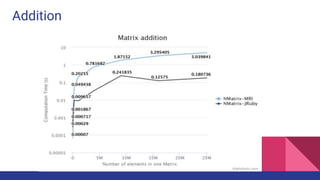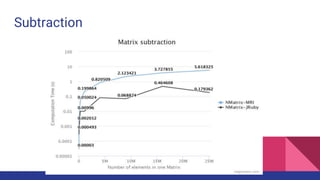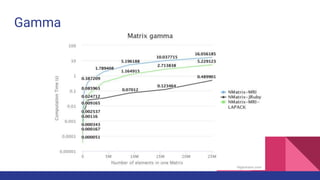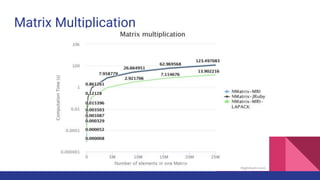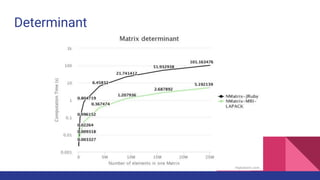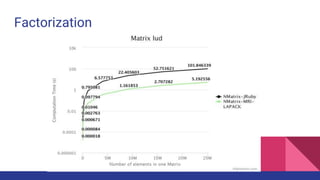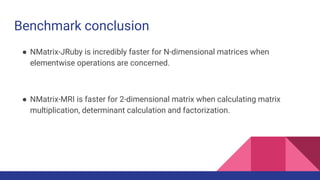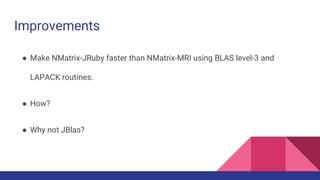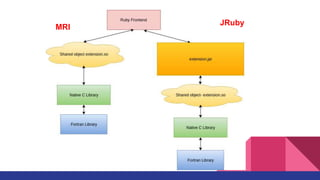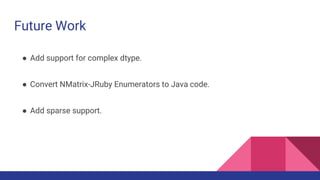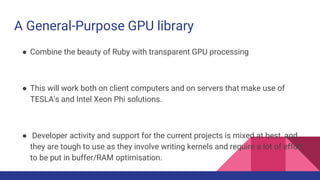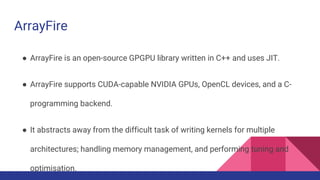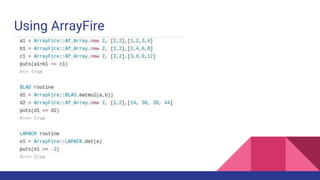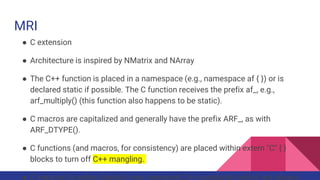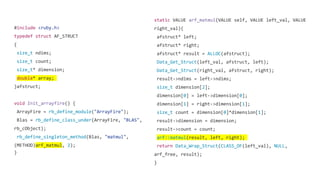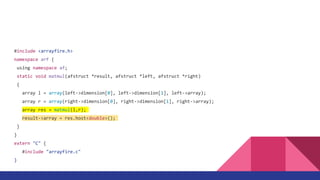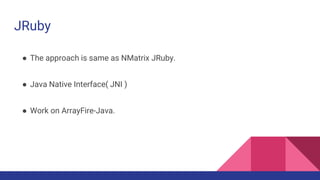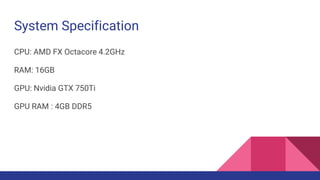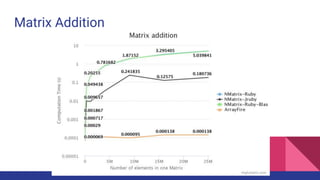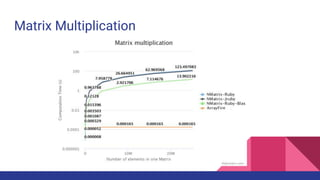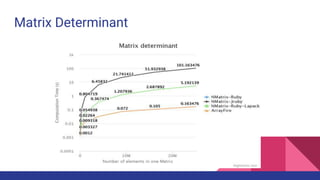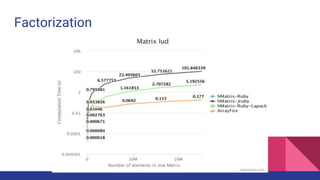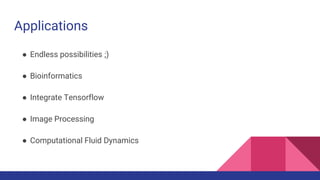1 of 70

### Fosdem2017 Scientific computing on Jruby

• 1. Scientific Computing on JRuby github.com/prasunanand
• 2. Objective ● A Scientific library is memory intensive and speed counts. How to use JRuby effectively to create a great tool/gem? ● A General Purpose GPU library for Ruby that can be used by industry in production and academia for research.
• 3. ● Ruby Science Foundation ● SciRuby has been trying to push Ruby for scientific computing. ● Popular Rubygems: 1. NMatrix 2. Daru 3. Mixed_models
• 4. NMatrix ● NMatrix is SciRuby’s numerical matrix core, implementing dense matrices as well as two types of sparse (linked-list-based and Yale/CSR). ● It currently relies on ATLAS/CBLAS/CLAPACK and standard LAPACK for several of its linear algebra operations.
• 6. Daru
• 7. Mixed_models
• 8. Nyaplot
• 9. SciRuby vs SciPy ● We love Ruby. ● We love Rails. ● Expressiveness of Ruby.
• 10. ● Known for performance JRuby is 10 times faster than CRuby. ● With truffle it’s around 40 times faster than CRuby. Truffle is supported by Oracle.
• 11. Say Hello!
• 12. NMatrix for JRuby ● Parallelism=> No Global Interpreter Lock as in case of MRI ● Easy Deployment(Warbler gem) ● Auto Garbage collection. ● Speed ● NMatrix for JRuby relies on Apache Commons Math
• 13. MDArray ● Not a unified interface for Sciruby gems=> Why not build a wrapper around MDArray ? ● MDArray is a great gem for Linear Algebra. ● MdArray used Parallel colt that was depreceated. ● However, every gem that used NMatrix as dependency needed to be reimplemented with MDArray.
• 14. How NMatrix works? ● N-Dimensional ● 2-Dimensional NMatrix
• 15. N-dimensional matrices are stored as a one-dimensional Array!
• 16. NMatrix Architecture MRI JRuby
• 17. N - dimensional Matrix
• 18. Elementwise Operation ● [:add, :subtract, :sin, :gamma] ● Iterate through the elements. ● Access the element; do the operation, return it
• 20. Challenges ● Autoboxing and Multiple data type ● Minimise copying of data
• 21. Errors that can’t be reproduced :p [ 0.11, 0.05, 0.34, 0.14 ] + [ 0. 21, 0.05, 0.14, 0.14 ] = [ 0, 0, 0, 0] ([ 0. 11, 0.05, 0.34, 0.14 ] + 5) + ([ 0. 21, 0.05, 0.14, 0.14 ] + 5) - 10 = [ 0.32, 0.1, 0.48, 0.28]
• 22. Autoboxing ● :float64 => double only ● Strict dtypes => creating data type in Java. Can’t Rely on Reflection ● @s = Array.new() ● @s = Java::double[rows*cols].new()
• 23. Autoboxing and Enumerators def each_with_indices nmatrix = create_dummy_nmatrix stride = get_stride(self) offset = 0 coords = Array.new(dim){ 0 } shape_copy = Array.new(dim) (0...size).each do |k| dense_storage_coords(nmatrix, k, coords, stride, offset) slice_index = dense_storage_pos(coords,stride) ary = Array.new if (@dtype == :object) ary << self.s[slice_index] else ary << self.s.toArray.to_a[slice_index] end (0...dim).each do |p| ary << coords[p] end yield(ary) end if block_given? return nmatrix end
• 24. Minimise copying of data ● Make sure you don’t make copies of data. ● Pass-by-Reference in action: ○ Use static methods as helpers.
• 25. 2 - dimensional Matrix
• 26. 2 - dimensional Matrix Operations ● [:dot, :det, :factorize_lu] ● In NMatrix-MRI, BLAS-III and LAPACK routines are implemented using their respective libraries. ● NMatrix-JRuby depends on Java functions.
• 27. Challenges ● Converting a 1-D array to 2-D array ● Array Size and Accessing elements ● Speed and Memory Required
• 29. Ruby Code index =0 puts Benchmark.measure{ (0...15000).each do |i| (0...15000).each do |j| c[i][j] = b[i][j] index+=1 end end } #67.790000 0.070000 67.860000 ( 65.126546) #RAM consumed => 5.4GB b = Java::double[15_000,15_000].new c = Java::double[15_000,15_000].new index=0 puts Benchmark.measure{ (0...15000).each do |i| (0...15000).each do |j| b[i][j] = index index+=1 end end } #43.260000 3.250000 46.510000 ( 39.606356)
• 31. Java Code public class MatrixGenerator{ public static void test2(){ for (int index=0, i=0; i < row ; i++){ for (int j=0; j < col; j++){ c[i][j]= b[i][j]; index++; } } } puts Benchmark.measure{MatrixGenerator.test2} #0.034000 0.001000 00.034000 ( 00.03300) #RAM consumed => 300MB public class MatrixGenerator{ public static void test1(){ double[][] b = new double; double[][] c = new double; for (int index=0, i=0; i < row ; i++){ for (int j=0; j < col; j++){ b[i][j]= index; index++; } } } puts Benchmark.measure{MatrixGenerator.test1} #0.032000 0.001000 00.032000 ( 00.03100)
• 32. Results Improves: ● 1000 times the speed ● 10times the memory
• 33. Mixed models ● After NMAtrix for doubles was ready, I tested it with mixed_models.
• 34. Benchmarking NMatrix functionalities
• 35. System Specifications ● CPU: AMD FX8350 0ctacore 4.2GHz ● RAM: 16GB
• 37. Subtraction
• 38. Gamma
• 39. Matrix Multiplication
• 40. Determinant
• 41. Factorization
• 42. Benchmark conclusion ● NMatrix-JRuby is incredibly faster for N-dimensional matrices when elementwise operations are concerned. ● NMatrix-MRI is faster for 2-dimensional matrix when calculating matrix multiplication, determinant calculation and factorization.
• 43. Improvements ● Make NMatrix-JRuby faster than NMatrix-MRI using BLAS level-3 and LAPACK routines. ● How? ● Why not JBlas?
• 44. MRI JRuby
• 45. Future Work ● Add support for complex dtype. ● Convert NMatrix-JRuby Enumerators to Java code. ● Add sparse support.
• 46. Am I done?
• 47. Nope!
• 48. Enter GPU
• 49. A General-Purpose GPU library ● Combine the beauty of Ruby with transparent GPU processing ● This will work both on client computers and on servers that make use of TESLA's and Intel Xeon Phi solutions. ● Developer activity and support for the current projects is mixed at best, and they are tough to use as they involve writing kernels and require a lot of effort to be put in buffer/RAM optimisation.
• 50. ArrayFire-rb ● Wraps ArrayFire library
• 51. ArrayFire ● ArrayFire is an open-source GPGPU library written in C++ and uses JIT. ● ArrayFire supports CUDA-capable NVIDIA GPUs, OpenCL devices, and a C- programming backend. ● It abstracts away from the difficult task of writing kernels for multiple architectures; handling memory management, and performing tuning and optimisation.
• 52. Using ArrayFire
• 53. MRI ● C extension ● Architecture is inspired by NMatrix and NArray ● The C++ function is placed in a namespace (e.g., namespace af { }) or is declared static if possible. The C function receives the prefix af_, e.g., arf_multiply() (this function also happens to be static). ● C macros are capitalized and generally have the prefix ARF_, as with ARF_DTYPE(). ● C functions (and macros, for consistency) are placed within extern "C" { } blocks to turn off C++ mangling. ● C macros (in extern blocks) may represent C++ constants (which are always
• 54. #include <ruby.h> typedef struct AF_STRUCT { size_t ndims; size_t count; size_t* dimension; double* array; }afstruct; void Init_arrayfire() { ArrayFire = rb_define_module("ArrayFire"); Blas = rb_define_class_under(ArrayFire, "BLAS", rb_cObject); rb_define_singleton_method(Blas, "matmul", (METHOD)arf_matmul, 2); } static VALUE arf_matmul(VALUE self, VALUE left_val, VALUE right_val){ afstruct* left; afstruct* right; afstruct* result = ALLOC(afstruct); Data_Get_Struct(left_val, afstruct, left); Data_Get_Struct(right_val, afstruct, right); result->ndims = left->ndims; size_t dimension; dimension = left->dimension; dimension = right->dimension; size_t count = dimension*dimension; result->dimension = dimension; result->count = count; arf::matmul(result, left, right); return Data_Wrap_Struct(CLASS_OF(left_val), NULL, arf_free, result); }
• 55. #include <arrayfire.h> namespace arf { using namespace af; static void matmul(afstruct *result, afstruct *left, afstruct *right) { array l = array(left->dimension, left->dimension, left->array); array r = array(right->dimension, right->dimension, right->array); array res = matmul(l,r); result->array = res.host<double>(); } } extern "C" { #include "arrayfire.c" }
• 56. JRuby ● The approach is same as NMatrix JRuby. ● Java Native Interface( JNI ) ● Work on ArrayFire-Java.
• 57. ● Place 'libaf.so' in the Load path. require 'ext/vendor/ArrayFire.jar' class Af_Array attr_accessor :dims, :elements def matmul(other) Blas.matmul(self.arr, other) end end
• 58. Benchmarking ArrayFire
• 59. System Specification CPU: AMD FX Octacore 4.2GHz RAM: 16GB GPU: Nvidia GTX 750Ti GPU RAM : 4GB DDR5